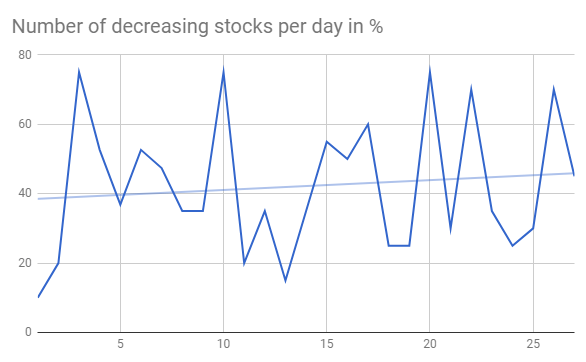# Prediction on Next Stock Market Correction

On Feb. 6, 2018, the stock market officially entered “correction” territory. A stock market correction is defined as a drop of at least 10% or more for an index or stock from its recent high.  During one week the stock data prices (closed price) were decreasing for many stocks. Are there any signals that can be used to predict next stock market correction?

I pulled historical data from 20 stocks selected randomly and then created python program that counts how many stocks (closed price) were decreased, increased or did not change for each day (comparing with previous day). The numbers then converted into percentage. So if all 20 stock closed prices decreased at some day it would be 100%. For now I was just looking at % of decreased stocks per day. Below is the graph for decreasing stocks. Highlighted zone A is when we many decreasing stocks during the correction.Number of decreasing stocks per day in %

### Observations

I did not find good strong signal to predict market correction but probably more analysis needed. However before this correction there was some increasing trend for number of stocks that close at lower prices. This is shown below. On this graph the trend line can be viewed as indicator of stock market direction.Number of decreasing stocks per day before correction in %

### Python Source Code to download Stock Data

Here is the script that was used to download data:

```from pandas_datareader import data as pdr
import time

# put below actual symbols as many as you need
symbols=['XXX','XXX', 'XXX', ...... 'XXX']

def get_data (symbol):

data = pdr.get_data_google(symbol,'1970-01-01','2018-02-19')
path="C:\\Users\\stocks\\"
data.to_csv( path + symbol+".csv")

return data

for symbol in symbols:
get_data(symbol)
time.sleep(7)
```

### Script for Stock Data Analysis

Here is the program that takes downloaded data and counts the number of decreased/increased/same stocks per day. The results are saved in the file and also plotted. Plots are shown after source code below.

And here is the link to the data output from the below program.

```# -*- coding: utf-8 -*-

import os

path="C:\\Users\\stocks\\"
from datetime import datetime
import pandas as pd
import numpy as np

def days_between(d1, d2):
d1 = datetime.strptime(d1, "%Y-%m-%d")
d2 = datetime.strptime(d2, "%Y-%m-%d")
print (d1)
print (d2)
return abs((d2 - d1).days)

i=10000   # index to replace date
j=20      # index for stock symbols
k=5       # other attributes
data = np.zeros((i,j,k))
symbols=[]

count=0

# get index of previous trade day
# because there is no trades on weekend or holidays
# need to calculate prvious trade day index instead
# of just subracting 1
def get_previous_ind(row_ind, col_count ):

k=1
print (str(row_ind) + "   " + str(col_count))
while True:
if  data[row_ind-k][col_count] == 1:
return row_ind-k
else:
k=k+1

if k > 1000 :
print ("ERROR: PREVIOUS ROW IS NOT FOUND")
return -1

dates=["" for i in range(10000) ]
# read the entries
listOfEntries = os.scandir(path)
for entry in  listOfEntries:

if entry.is_file():
print(entry.name)
stock_data = pd.read_csv (str(path) + str(entry.name))
symbols.append (entry.name)

for index, row in stock_data.iterrows():
ind=days_between(row['Date'], "2002-01-01")

dates[ind] = row['Date']
data[ind][count] = 1
data[ind][count] = row['Close']

if (index > 1):
print(entry.name)
prev_ind=get_previous_ind(ind, count)
delta= 1000*(row['Close'] - data[prev_ind][count])
change=0
if (delta > 0) :
change = 1
if (delta < 0) :
change = -1
data[ind][count] = change
data[ind][count] = 1

count=count+1

upchange=[0 for i in range(10000)]
downchange=[0 for i in range(10000)]
zerochange=[0 for i in range(10000)]
datesnew = ["" for i in range(10000) ]
icount=0
for i in range(10000):
total=0
for j in range (count):

if data[i][j] == 1 :
datesnew[icount]=dates[i]
total=total+1
if (data[i][j] ==0):
zerochange[icount]=zerochange[icount]+1
if (data[i][j] ==1):
upchange[icount]=upchange[icount] + 1
if (data[i][j] == - 1):
downchange[icount]=downchange[icount] + 1

if (total != 0) :
upchange[icount]=100* upchange[icount] / total
downchange[icount]=100* downchange[icount] / total
zerochange[icount]=100* zerochange[icount] / total
print (str(upchange[icount]) + "  " +  str(downchange[icount]) + "  " + str(zerochange[icount]))
icount=icount+1

df=pd.DataFrame({'Date':datesnew, 'upchange':upchange, 'downchange':downchange, 'zerochange':zerochange })
print (df)
df.to_csv("changes.csv", encoding='utf-8', index=False)

import matplotlib.pyplot as plt

downchange=downchange[icount-200:icount]
upchange=upchange[icount-200:icount]
zerochange=zerochange[icount-200:icount]

# Two subplots, the axes array is 1-d
f, axarr = plt.subplots(3, sharex=True)
axarr.plot(downchange)
axarr.set_title('downchange')
axarr.plot(upchange)
axarr.set_title('upchange')
axarr.plot(zerochange)
axarr.set_title('zerochange')
plt.show()
```Number of stocks increasing decreasing same in %